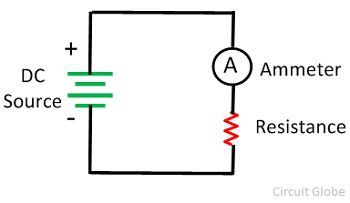# Difference Between Galvanometer & Ammeter

The most significant difference between the galvanometer and ammeter is that the galvanometer show both the direction and the magnitude of the current, whereas the ammeter only shows the magnitude of the current. The other differences between the galvanometer and the ammeter are shown below in the comparison chart.

The galvanometer uses a moving coil which is free to rotate between the permanent magnet. When the current flows through the coil, it becomes deflected. The deflection of the coil is directly proportional to the current flow through it. The galvanometer is converted into the ammeter by connecting the resistance in parallel with the circuit. And if the resistance is connected in series with a galvanometer, then it is used as a voltmeter.

The ammeter is also known as the ampere meter. The ampere is the unit of the current, so the ampere meter is the type of meter which measures the magnitude of current passes through it. It is connected in series with the circuit for determining the exact value of circuit current.

## Content: Galvanometer Vs Ammeter

### Comparison Chart

Basis for Comparison Galvanometer Ammeter
DefinitionInstrument use for detecting the strength and direction of small current presence in a circuit. Instrument used for determining the magnitude of current presence in the electrical circuit
SymbolDirection of current Shows Do not shows.
Type of Device Mechanical Mechanical or Electronic.
Magnetic Field Requires Work with or without magnetic field.
Accuracy LessMore
Measuring Current Measures only direct current Measures both direct and alternating current.
Sensitive
More Less
Applications In bridge and potentiometer measurements.In an electrical circuit.

### Definition of Galvanometer

The galvanometer is the current measuring instrument which is mostly used in bridges and potentiometer for showing the zero current. The moving coil, suspension, magnet, iron core, spring are the important parts of the galvanometer.

The galvanometer has permanent magnets between which the coil of wire is placed. When the current passes through the coil, the magnetic field induces across the wire. The magnetic field of coil cuts the magnetic field of permanent magnet due to which the force is exerted on the coil, and it starts moving.At the end of the coil, the needle is attached whose deflection shows the presence of current. The deflection of the needle is directly proportional to the magnitude of current flows through it.

### Definition of Ammeter

The ammeter is an electronic device which is connected in series with the circuit for measuring the current flows through it. The construction of the galvanometer is same as that of the ammeter the only difference is that the ammeter has additional resistance connected in parallel with the circuit.The low resistance wires are used in the ammeter so that the whole circuit current passes through it.

## Key Differences between Galvanometer and Ammeter

The following are the key differences between the galvanometer and the ammeter.

1. The galvanometer is the mechanical devices used for determining the magnitude as well as the direction of the current, whereas the ammeter is the electric devices used for measuring the magnitude of the current.
2. The galvanometer shows the direction of current flows in the circuit whereas the ammeter measures the magnitude of current flows through it.
3. The magnetic field is required for the working of galvanometer whereas it is not essential for the ammeter.
4. The accuracy of the galvanometer is less as compared to the ammeter.
5. The galvanometer is only used for measuring the direct current, whereas the ammeter is used for measuring the both the direct and alternating current.
6. The sensitivity of the galvanometer is more as compared to the ammeter.
7. The galvanometer is mostly used in bridge and potentiometer for knowing the zero current whereas the ammeter is directly connected in series with the circuit whose magnitude is to be measured.

### Conclusion

The galvanometer and ammeter both are the measuring devices. The ammeter is the implemented form of the galvanometer which shows the accurate value of current passes through it in milliamperes or amperes.